# Trevor

Trevor wishes to tile the floor of his bathroom. The bathroom is rectangular and is 4.2m long and 3.3m wide. The tiles are 30cm by 30cm. Calculate the cost to tile the floor if each tile costs 72 cents.

p =  110.88 USD

### Step-by-step explanation: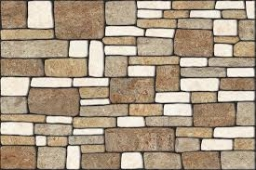Did you find an error or inaccuracy? Feel free to write us. Thank you!Tips to related online calculators
Do you want to convert area units?

#### You need to know the following knowledge to solve this word math problem:

We encourage you to watch this tutorial video on this math problem:

## Related math problems and questions:

• The corridor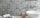The corridor is 12 m long and 3.6 m wide. It must be paved with rectangular tiles measuring 15 cm and 30 cm. Are 1000 pieces of tiles enough to pave the corridor?
• Flooring a roomFind the cost of flooring a room 6.5 m by 5 m with square tiles of sides 25 cm at the rate of rupees 9.40 per tile.
• Pool tilesThe pool is 25m long, 10m wide, and 160cm deep. How many m2 of tiles will be needed on the walls and the pool? How many tiles are needed when 1 tile has a square shape with a 20cm side? How much does it cost when 1m2 of tiles costs 258 Kc?
• Bathroom 2A bathroom is 2.4 meters long and 1.8 meters wide. How many square tiles 1 dm on each side are to be used to cover it?
• Tiles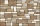The rectangular floor of the 6 x 1.8 m dimensions is to be covered with 50 cm square tiles. How many tiles will be needed?
• Bathroom 3De Mesa family will soon be occupying their newly renovated house . However the bathroom, measuring 10ft by 16ft, still to be covered with tiles . If the tiles that they desire measures 2/5 ft by 2/5ft, how many tiles will they need to cover the bathroom
• Tiles path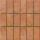A rectangular park measures 24mX18m. A path 2.4m wide is constructed all around it. Find the cost of paving the path with cement tiles of size 60cm X 40cm at the rate of \$5.60 per tile ?
• Bathroom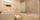How many CZK do we pay for lining the perimeter walls of the bathroom with rectangular shape with dimensions 3.5 m and 4 m, high 1.5 m if 1 square m tile cost 300 CZK?
• Pavilion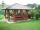The rectangular pavilion with dimensions 3.5 m and 2.75 m to be paved with square tiles of side 25 cm price of CZK 22 per 1 piece or rectangular tiles with sides of 20 cm and 15 cm in the price of CZK 11 per 1 pc. Which solution is cheaper (write its pric
• Tiles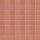How many 46 cm square tiles will cover a floor 22.08 m by 8.74 m?
• Decorative fenceThe rectangular garden is 57 m long and 42 m wide. Calculate how much m² it will reduce if it encloses with a 60 cm wide decorative fence.
• PaintingThe room is long 50 meters and wide 60dm and 300 cm high. Calculate how much it will cost painting if area of windows and doors is 15% of the total area. One square meter cost 50cents.
• Square tilesThe room has dimensions of 12 meters and 5.6 meters. Determine the number of square tiles and their largest dimension to exactly cover the floor.
• GardenThe rectangular garden has a length 99 m and width 84 m. Calculate how many m2 will decrease its area if land by the ornamental fence with width 30 cm.
• The carpet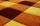How many meters of carpet 90 cm wide need to cover floor room which has a rectangular shape with a lengths 4.8 m and 2.4 m if the number of pieces on the carpet is needed to be lowest?
• If one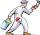If one litre of pant covers an area of 5 m2 how much paint is needed to cover: a) rectangular swimming pool With dimensions 4m x 3m x 2.5m (the Inside walls and the floor only) b) the Inside walls and floor of a cylindrical reservoir with diameter 3m and
• Glass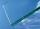Peter broke the window glass with size 110 cm and 90 cm. 1 square meter of glass costs 11 USD. How much money is need to pay for a new glass?